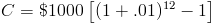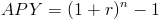Custom Search

# Compound Interest

Compound Interest Calculator (Click Here or Scroll Down)The compound interest formula calculates the amount of interest earned on an account or investment where the amount earned is reinvested. By reinvesting the amount earned, an investment will earn money based on the effect of compounding.

Compounding is the concept that any amount earned on an investment can be reinvested to create additional earnings that would not be realized based on the original principal, or original balance, alone. The interest on the original balance alone would be called simple interest. The additional earnings plus simple interest would equal the total amount earned from compound interest.

### Rate and Period in Compound Interest Formula

The rate per period (r) and number of periods (n) in the compound interest formula must match how often the account is compounded. For example, if an account is compounded monthly, then one month would be one period. Likewise, if the account is compounded daily, then one day would be one period and the rate and number of periods would accommodate this.

#### Example of Compound Interest Formula

Suppose an account with an original balance of \$1000 is earning 12% per year and is compounded monthly. Due to being compounded monthly, the number of periods for one year would be 12 and the rate would be 1% (per month). Putting these variables into the compound interest formula would showThe second portion of the formula would be 1.12683 minus 1. By multiplying the original principal by the second portion of the formula, the interest earned is \$126.83.

##### Simple Interest vs. Compound Interest

Using the prior example, the simple interest would be calculated as principal times rate times time. Given this, the interest earned would be \$1000 times 1 year times 12%. After using this formula, the simple interest earned would be \$120. Using compound interest, the amount earned would be \$126.83. The additional \$6.83 earned would be due to the effect of compounding. If the account was compounded daily, the amount earned would be higher.

###### Compound Interest Formula in Relation to APY

The compound interest formula contains the annual percentage yield formula ofThis is due to the annual percentage yield calculating the effective rate on an account, based on the effect of compounding. Using the prior example, the effective rate would be 12.683%. The compound interest earned could be determined by multiplying the principal balance by the effective rate.

###### Alternative Compound Interest Formula

The ending balance of an account with compound interest can be calculated based on the following formula:As with the other formula, the rate per period and number of periods must match how often the account is compounded.

Using the prior example, this formula would return an ending balance of \$1126.83.

New to Finance?